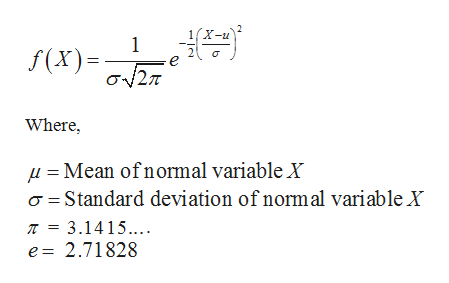In a standard normal distribution, what is the area under the graph for z>0? Explain.

Question

In a standard normal distribution, what is the area under the graph for z>0? Explain.

Step 1

Normal distribution:

A random variable X is said to follow a normal distribution if the probability distribution is as follows:help_outlineImage Transcriptionclose1(X-u 1 f(X)2 Where Mean of normal variable X aStandard deviation of normal variable X T = 3.1415.. e 2.71828 fullscreen
Step 2

Standard Normal Distribution:

A random variable z is said to be a standard normal variable if z follows normal distribution with mean µ = 0 and variance σ2 =1. The formula for the probability distribution of z is,

Step 3

Area under the graph for z >0:

The standard normal distribution is symmetric about its mean, z = 0. Also, the total area under the standard normal probability distribution equals 1. Taken together, these two factors imply that the areas ...

Want to see the full answer?

See Solution

Want to see this answer and more?

Our solutions are written by experts, many with advanced degrees, and available 24/7

See Solution
Tagged in

Other